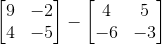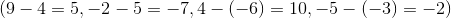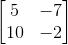# Precalculus : Matrices and Vectors

## Example Questions

1 2 10 11 12 13 14 15 16 18 Next →

### Example Question #9 : Find The Difference Of Two MatricesTo solve this problem, subtract the corresponding numbers in each matrix:. Then, put those answers in the corresponding places in the answer matrix, so that you get:.